# SAT Math Multiple Choice Question 793: Answer and Explanation

### Test Information

Question: 793

13.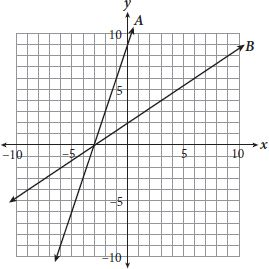If the equation of line A shown on the graph is given by y = mx + b, and the equation of line B is given by y = k(mx + b), what is the value of k?

• A.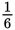• B.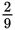• C. 6
• D. 9

Getting to the Answer: First, determine the equation of line A. Remember that slope is equal to rise over run, so count the change in y and the change in x from one point on the line to the next. The slope is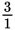or just 3. Next, find the y-intercept: 9. Now, find the equation of line B: The slope is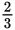, and the y-intercept is 2. So, the equations are: Line A: y = 3x + 9 and Line B: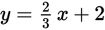. Notice that the numbers in the equation of line A are larger, so k must be a fraction, which means you can eliminate C and D. To choose between A and (B), multiply the number by the equation of line A to see if it matches the equation of line B. Because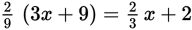(the equation of line B),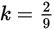. Therefore, (B) is correct.